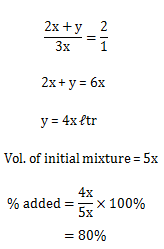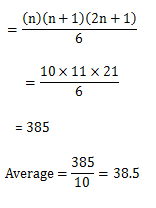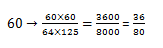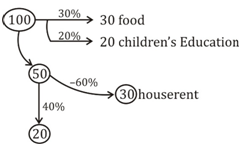# SSC Quantitative Aptitude Questions (Day-79)

Dear Aspirants, you can find the Quantitative Aptitude questions with detailed explanations for the SSC exams. Nowadays the competitive level of the exam has been increasing consistently. Due to the great demand for the government job, the level of the toughness reached greater. Candidates have to enhance the preparation process in order to drive in the right path. It doesn’t need to clear the prescribed cutoff. You must have to score good marks more than the cut off marks to get into the final provisional list. Here we have updating the Quantitative Aptitude questions with detailed explanations on a daily basis. You can practice with us and measure your level of preparation. According to that you can sculpt yourself in a proper way. SSC aspirants kindly make use of it and grab your success in your career.

Start Quiz

1) In a mixture, milk and water are in ratio of 2: 3. Some milk is added to the mixture because of which ratio of milk and water becomes 2 : 1. How muchmilk was added as a percentage of initial mixture?

(a) 75

(b) 60

(c) 80

(d) 50

2) The average of marks obtained by A and B is 15 less than that of average marks obtained by B and C. If the marks obtained by C is 65, what is themarks obtained by
A?

(a) 35

(b) 50

(c) 65

(d) 80

3) What is the average of squares of first 10 natural numbers?

(a) 55.5

(b) 45.6

(c) 38.5

(d) 40.5

4) Cost price and selling price of an article are in ratio 13 : 9. If the loss incurred on article is Rs 320, what is the sum (in Rs) of cost and selling prices?

(a) 1480

(b) 1620

(c) 1500

(d) 1760

5) By selling 64 apples for Rs 60 a person gains 25%. In order to have 40% loss, how many apples shall he sell for Rs 36?

(a) 80

(b) 70

(c) 60

(d) 50

6) A mixture contains 18% copper by weight. How much mixture (in kg) is required to obtain 81 kg of copper?

(a) 350

(b) 300

(c) 450

(d) 250

7) A person spends 30% of his income on food, 20% on children’s education and 60% of remaining on house rent. What percent of income is left withhim?

(a) 20

(b) 30

(c) 35

(d) 25

8) A man rides his vehicle at the rate of 36 km/hr but stops for 12 minutes, to change parts at the end of every 14th km. What will be the time taken tocover a distance of 90 km?

(a) 6 hour 12 minutes

(b) 2 hours 30 minutes

(c) 3 hours 42 minutes

(d) 5 hours 32 minutes

9) A walks at a uniform speed of 8 km/hr and 8 hours after his start, B starts on his cycle after him at speed of 24 km/hr. How far (in km) from thestarting point will B catch A?

(a) 72

(b) 96

(c) 120

(d) 144

10) What is the rate (in percent) of simple interest at which a sum of money becomes three times of itself in 50 years?

(a) 2

(b) 4

(c) 5

(d) 8Avg. of B & C ⇒ x; Total of B & C = 2x

Avg. of A & B ⇒ (x – 15); Total of A & B = 2(x – 15)

Difference b/w both the totals ⇒ (A + B) – (B + C)

= 2x – 30 – 2x

A – C = – 30

A – 65 = – 30

A = 35

Sum of squares of first 10 natural nos.Given ⇒ 13x – 9x = 4x = 320

x = 80

C.P. + S.P. = 13x + 9x

= 22x

=1760

125→ 60/64Hence for 40% loss he will sell 80 apples for Rs 36.

18% copper by weight means,

∵  For 18 Kg of copper ⇒ 100 Kg of mixture is required.

∴  for 81 Kg of Copper,mixture required = (100/18) * 81 = 450KgAs the bus is stopping at each 14th Km then total no of stoppage = 90/14 = 6.xx= 6No. of stoppage

Time wasted in 6 no of stoppages = 12*6 = 72 minutes

Time required to travel 90 Km without stoppage = 90/36 = 2 hour 30 minutes

Total time required to travel with stoppages       = 2hour 30 minutes + 1 hour 12 minutes

= 3 hour 42 minutes

Initial difference = 8 × 8 = 64 km

Total relative speed = 16 kmph

Total time required to catch = 64/16 = 4 hrs.

Total distance = 64 + 8 × 4 = 96 kms